American Journal of Modern Physics
Volume 5, Issue 1-1, February 2016, Pages: 18-22

Inherent Contradictions in Higgs Boson Theory

Eliyahu Comay

Charactell Ltd., Tel Aviv, Israel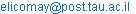(E. Comay)

Eliyahu Comay. Inherent Contradictions in Higgs Boson Theory. American Journal of Modern Physics. Special Issue: Physics Without Higgs and Without Supersymmetry. Vol. 5, No. 1-1, 2015, pp. 18-22. doi: 10.11648/j.ajmp.s.2016050101.13

Abstract: The analysis relies on several general principles such as the Variational Principle and the broader sense of Bohr's Correspondence Principle. These principles together with other elements of theoretical physics provide a set of restrictions by which every quantum theory must abide. The paper proves that the Higgs boson theory contains many contradictions that undermine its physical validity. It is also explained why the 125 GeV particle H0 which was recently found at CERN cannot be a Higgs boson.

Keywords: The Higgs Boson, Quantum Field Theory, the Variational Principle, the Bohr's Correspondence Principle

1. Introduction

The existence of a Higgs boson has been suggested quite a long time ago [1,2]. This particle is now a part of the Standard Model (SM). Besides an electrically neutral Higgs boson (denoted H0), the possibility of the existence of charged Higgs bosons (denoted H±) and of doubly charged Higgs bosons (denoted H±±) is also mentioned in the literature . The main objective of this work is to use well established principles of physics and examine the structure of the Higgs boson theory. The analysis relies on special relativity, Maxwellian electrodynamics and on fundamental elements of quantum field theory (QFT). The work uses the principal element of theoretical physics, which states that a physical theory must have an error free mathematical structure. It is shown below that far-reaching consequences are derived from this principle.

This work uses standard notation and the metric is diag (1,-1,-1,-1). Units where ħ = c = 1 are used.

The analysis applies several specific criteria which are explained in the second section. Additional requirements that any quantum theory must satisfy are derived in the third section. Many failures of the Higgs boson theory are described in the fourth section. The fifth section shows further contradictions of a charged Higgs boson. The last section contains concluding remarks.

2. Fundamental Principles

Evidently, the demand that a physical theory must be error-free provides a constraint that every acceptable physical theory must satisfy. Alternatively, a theory that fails to do that can be rejected without any further examination of its details.

Physics is a mature science and some of its elements have acquired an indisputable status. Here the validity of Special relativity and of Maxwellian electrodynamics is used as cornerstones of the analysis and an inconsistency of a given theory with an element of either of them justifies the rejection of the examined theory.

The form of the electromagnetic interaction is obtained from the following covariant substitution (see p. 84 of  and pp. 47-49 of )

pμ → pμ ‒ eAμ.              (1)

This form of the electromagnetic interaction is used herein as a requirement that any theory of an electrically charged particle must satisfy.

Another element which is used here is the broader sense of Bohr's correspondence principle, as stated by the present version of Wikipedia: "The term is also used more generally, to represent the idea that a new theory should reproduce the results of older well-established theories (which become limiting cases) in those domains where the old theories work." The relationships between relativistic mechanics and Newtonian mechanics and between quantum mechanics and classical physics are convincing examples of the Bohr's correspondence principle. A clear discussion of this subject can be found on pp. 1-6 of Rohrlich's book . The special cases of the correspondence between quantum mechanics and QFT and of the relation between quantum theories and classical physics are used below.

The de Broglie relationship between the energy-momentum of a massive particle and its wave attributes state that

E = ħω                      (2)

(see pp. 48-49 of ). This is another fundamental principle which is used below.

Other criteria which have been published few years ago  are not so well known. Therefore, for the clarity of the analysis they are described briefly herein.

Consider a two slit interference pattern of a massive quantum particle. A point where the interference is totally destructive is explained by a phase difference of π. The phase is an argument of an analytic function exp(iφ) which can be expanded in an infinite power series. Therefore, the phase must be a dimensionless Lorentz scalar. This goal can be achieved if the phase is the action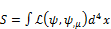(3)

and the Lagrangian density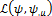is a Lorentz scalar whose dimension is [L‒4]. As a matter of fact, the application of a Lagrangian density as a cornerstone of a quantum theory is widely used in the literature (see [9,10] as well as many other textbooks). The action is a quantity used in an application of the variational principle. Therefore, the equation of motion of the quantum particle is the Euler-Lagrange equation of the Lagrangian density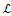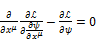(4)

The field function of a quantum particle and its derivatives are used as factors in appropriate terms of the Lagrangian density. Therefore, the dimension [L‒4] of the Lagrangian density means that the field function acquires dimension.

Another issue is the mathematical form of the field function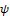(xμ), which depends on a single set of four space-time coordinates xμ. It means that the QFT field function describes an elementary pointlike particle. Indeed, a description of a particle that has a structure must define the location of its center and the specific distribution of the particle around its center. Evidently, for the second task the field function must depend on other parameters. For example, the π+ particle has a charge radius of about 0.67 fm . And indeed, the π+ comprises a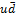quark-antiquark pair. Hence, its state is described by two independent parameters (xμ, xν ), where each of which is related to one member of this pair. Analogous conclusions hold for the π0. Therefore, pions are not Yukawa particles because the Yukawa function ϕ(xμ) depends on a single set of four space-time coordinates xμ (see p.213 of ).

The discussion presented in the rest of this work illustrates the powerful meaning of the principles and the criteria described above. Their role in an evaluation of the acceptability of a given theory is analogous to that of conservation laws. For example, if a given theory predicts a process whose final result is inconsistent with energy conservation then it is rejected right away.

3. Specific Requirements

One can use the principles and the criteria described in the second section and obtain additional requirements that must be satisfied by any given physical theory of a quantum particle.

Ÿ In classical physics energy is a well defined quantity. Hence, the broader sense of Bohr's correspondence principle means that quantum mechanics and QFT must have a self-consistent expression for energy. The energy operator is called Hamiltonian. The following well known process illustrates the importance of this issue . Let us examine an inelastic scattering event. The chronological order of this process is as follows:

a.   First, two particles move in external electromagnetic fields. Relativistic classical mechanics and classical electrodynamics describe the motion.

b.   The two particles are very close to each other. Relativistic Quantum Mechanics (RQM) describes the process.

c.   The two particles collide and interact. New particles are created. QFT describes the process.

d.   Particle creation ends but they are still very close to one another. RQM describes the state.

e.   Finally, the outgoing particles depart. Relativistic classical mechanics and classical electrodynamics describe the motion.

The energy conservation law tells that the energy of the outgoing particles equals the energy of the incoming particles. This effect plays a fundamental role in the analysis of collision data. Hence, RQM and QFT must have a self-consistent expression for energy in order to transfer this information from the incoming particles to the outgoing particles.

The Hamiltonian is the energy operator. Therefore, it is a 0-component of a 4-vector.

Density is also a 0-component of a 4-vector (see p. 75 of ). Hence, the Hamiltonian density is a 00-component of a second rank tensor. The 00-component of this tensor is obtained from the Lagrangian density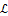by means of the well known Legendre transformation (see pp. 5-16 of )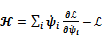(5)

where the index i runs on all functions.

Ÿ The de Broglie relationship means that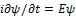. On the other hand the Hamiltonian is the energy operator. Hence, one obtains the fundamental equation of quantum mechanics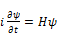(6)

On the other hand the analysis relies on the variational principle and the Euler-Lagrange equation (4) of the specific Lagrangian density is another equation of motion of the system. Thus, one obtains the following requirement: The Euler-Lagrange equation of the particle's Lagrangian density must agree with the fundamental quantum mechanical equation (6).

It can be concluded that each subject discussed in the second and in the third sections yields a theoretical constraint that every acceptable quantum theory must satisfy. These constraints are applied below.

4. Theoretical Physics vs. the Higgs Boson

The discussion relies on the structure of the Lagrangian density of the Higgs boson. This Lagrangian density can be written in the following compact form (see p. 715 of )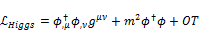(7)

where ϕ is a scalar function of the Higgs boson, m denotes the Higgs mass and OT denotes other terms whose specific form is not discussed herein. (The sign of the mass term of (7) is irrelevant to the discussion.) The first term of (7) is the highest power of the derivatives of ϕ and OT consists of terms that contain a lower power of the derivatives.

1.   As found above, the dimension of the Lagrangian density is [L‒4]. Therefore, the first term of (7) proves that the dimension of the Higgs function ϕ is [L‒1]. The density of a Schrödinger particle is written in terms of the wave function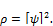(8)

It is well known that the Schrödinger density (8) satisfies the continuity equation (see p. 54 of ). Hence the dimension of the Schrödinger function is [L‒3/2]. The dierent dimension of the Higgs and the Schrödinger functions means that the Higgs theory has no non-relativistic limit. For this reason it is inconsistent with the broader sense of the Bohr's correspondence principle which is mentioned in the second section.

2.   The Higgs function ϕ is a scalar function whose dimension is [L‒1]. Hence, the product ϕϕ is a scalar whose dimension is [L‒2]. On the other hand, density is a 0-component of a 4-vector whose dimension is [L‒3] (see p. 75 of ). Hence, the product ϕϕ does not represent the density of the Higgs boson. This result is related to the previous contradiction. For having an expression for density one may examine a combination of time derivatives of factors of the product ϕϕ. However, in this case the following contradictions arise. The construction of the Hilbert space requires a well defined expression for density. In the Heisenberg picture the wave functions are time-independent (see pp. 7-8, 11-12, 130 of ). It means that in the Heisenberg picture a time-derivative of the Higgs function vanishes identically and the Hilbert space and its associated Fock space cannot be constructed. Therefore, the operators of the Higgs field, which operate on the Fock space become meaningless. By the same token, solutions of the Schrödinger equation can be used for constructing a Hilbert space of the Heisenberg time-independent wave functions. This is another inconsistency of the Higgs theory with the Bohr's correspondence principle.

3.   Let us examine the Euler-Lagrange equation of the Higgs boson. Applying (4) to the Higgs Lagrangian density (7), one finds the following equation for ϕ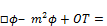(9)

Here the d'Alembertian operator is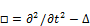.

It means that the Euler-Lagrange equation of the Higgs boson is a second order differential equation with respect to the time variable. On the other hand, the fundamental equation of quantum mechanics (6) is a first order differential equation with respect to the time variable. Hence, these equations are not identical (see p. 35 of ) and the requirement obtained near the end of section 3 is violated.

4.   Let us have a look at the Higgs Hamiltonian density. Applying (5) to (7), one obtains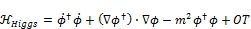(10)

As shown above, each term of the Higgs density must have just one factor of the form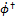or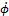. Therefore, the first term of (10) proves that the Higgs Hamiltonian must depend on a time-derivative of the Higgs function ϕ. This is yet another inconsistency of the Higgs theory with the broader sense of the Bohr's correspondence principle, because the Schrödinger Hamiltonian is independent of time derivatives of the wave function.

5.   The Lagrangian density of a given field can be used for constructing the energy-momentum tensor. Here one obtains (see p. 83 of )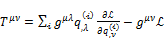(11)

where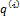denotes the ith independent coordinate. Substituting the Higgs Lagrangian density (7) into (11), one finds that the Higgs energy-momentum tensor has a term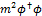. It means that the Higgs energy-momentum tensor depends quadratically on its mass m. On the other hand the classical energy-momentum tensor depends linearly on the mass (see p. 92 of ). Here one sees yet another contradiction of the Higgs theory with the broader sense of the Bohr's correspondence principle. Indeed, the energy-momentum tensor is used in Einstein's equations of general relativity. It means that the Higgs mass is an inherently different kind of mass whose physical properties are inconsistent with those of ordinary mass.

6.   The Higgs Lagrangian density (7) has the term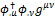which is also found in the Klein-Gordon (KG) Lagrangian density (see , p. 26). The KG density is derived from this quantity (see p. 193 of )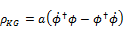(12)

where a denotes a constant factor. This expression is antisymmetric with respect to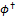, ϕ. On the other hand, the KG Hamiltonian density has the term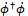which is symmetric in, ϕ (see p. 192 of ). Hence, density cannot be extracted from the KG Hamiltonian density. It means that the KG equation has no explicit differential form for its Hamiltonian .

The same result is obtained for the Higgs boson because its Lagrangian density (7) has the same term. By contrast, the Schroedinger equation has an explicit differential operator for its Hamiltonian. This is yet another inconsistency of the Higgs theory with the broader sense of the Bohr's correspondence principle.

5. Problems with the Charged Higgs Boson

The existence of charged and of doubly charged Higgs bosons is considered in the literature . Evidently, all contradictions of the neutral Higgs boson which are discussed in the previous section also hold for a charged Higgs boson. The following lines prove that new problems arise for a charged Higgs boson.

The second item of the previous section explains why the Higgs density must contain a derivative of its function ϕ. Since density is a 0-component of a 4-vector, one finds that also the Higgs 4-current must depend on a derivative. An application of (1) means that the Higgs density depends on the electromagnetic 4-potential Aμ .

An examination of the full Lagrangian density clarifies the problem. This quantity consists of three parts: the matter Lagrangian density, the electromagnetic fields' part and the interaction term (see p. 75 of ). Therefore, the results must abide by the laws of Maxwellian electrodynamics. The interaction term of Maxwellian fields with charged matter takes the form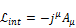(13)

where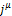is the 4-current of the charged matter. It means that the electromagnetic interaction of a charged Higgs boson has a term which is quadratic in Aμ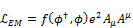(14)

where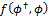is a function of the Higgs fields. The following lines explain why the quite unusual interaction term (14) is physically unacceptable.

Ÿ The interaction strength of the unusual term of (14) is proportional to the square of the electric charge e2 whereas the interaction strength of Maxwellian fields with an electrically charged matter is proportional to the electric charge e.

Ÿ The unusual term of (14) depends quadratically on the 4-potentials Aμ whereas Maxwellian interaction depends linearly on the 4-potentials.

It is well known that the inhomogeneous pair of Maxwell equations is obtained from a variation of the electromagnetic fields and their 4-potentials. Here the charged matter is assumed to be given (see pp. 78-80 of ). The calculation assumes that the interaction term of the Lagrangian density is linear in the 4-potentials Aμ and it is also proportional to the electric charge e. Hence, the electrically charged Higgs boson is inconsistent with Maxwellian electrodynamics. It can be concluded that a comparison with the neutral Higgs boson shows that an electrically charged Higgs boson also suffers from some specific contradictions that stem from the indisputable laws of Maxwellian electrodynamics.

6. Concluding Remarks

Sections 2 and 3 of this work show how general physical principles provide a list of powerful constraints that facilitate the examination of the acceptability of quantum theories. This approach is applied to the Higgs Lagrangian density and shows that many contradictions arise. For this reason, the experimentally detected 125 GeV H0 particle cannot be a Higgs boson, simply because a particle cannot be explained by a theory that is full of contradictions. It is interesting to note that as of January 2015 the Particle Data Group, which is the authorized organization for the determination of the existence of particles and of their properties, still has certain reservations about the identification of the H0 particle as a Higgs boson . Many other sceptical opinions on this identification of the H0 particle can be found on the web.

The Higgs boson equation (9) is an example of a second order quantum theory. As a matter of fact, apart from the sign of the mass term and the existence of lower order terms, the highest order term of its time-derivatives is a part of this expression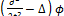. It follows that the Higgs equation belongs to the KG family. It is interesting to note Dirac's lifelong objection to these equations. He said on this issue: "I found this development quite unacceptable. It meant departing from the fundamental ideas of the non-relativistic quantum mechanics, ideas which demanded a wave equation linear in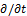. It meant abandoning the whole beautiful mathematical scheme for the sake of introducing certain physical ideas" (see pp. 3-4 of ). The present work provides further arguments that support Dirac's opinion.

Mathematics and physics go hand in hand for several centuries. The profound role of mathematics in the structure of physical theories is agreed by all physicists  (and by all engineers who use it for designing magnificent devices). The quite large numbers of contradictions of the Higgs boson theory which are described in this work make a basis for the expectation that this theory will be abandoned.

References

1. P. W. Higgs, Phys. Rev. Lett., 13, 508 (1964).
2. F. Englert and R. Brout, Phys.Rev. Lett., 13, 321 (1964).
3. K.A. Olive et al. (Particle Data Group), Chin. Phys. C38, 090001 (2014) (URL: http://pdg.lbl.gov)
4. J. D. Bjorken and S.D. Drell, Relativistic Quantum Fields (McGraw-Hill, New York, 1965).
5. L. D. Landau and E. M. Lifshitz, The Classical Theory of Fields (Elsevier, Amsterdam, 2005).
6. F. Rohrlich, Classical Charged Particle, (World Scientific, New Jersey, 2007).
7. L. D. Landau and E. M. Lifshitz, Quantum Mechanics (Pergamon, London, 1959).
8. E. Comay, Prog. in Phys., 4, 91 (2009).
9. S. Weinberg, The Quantum Theory of Fields, Vol. I, (Cambridge University Press, Cambridge, 1995).
10. M. E. Peskin and D. V. Schroeder, An Introduction to Quantum Field Theory(Addison-Wesley, Reading Mass., 1995).
11. J. D. Bjorken and S.D. Drell, Relativistic Quantum Mechanics (McGraw-Hill, New York, 1964).
12. E. Comay, Prog. in Phys. 4, 9 (2012).
13. G. F. Carrier and C. E. Pearson, Partial Differential Equations(Academic,Boston, 1988).
14. W. Pauli and V. Weisskopf, Helv. Phys. Acta, 7, 709 (1934). English translation: A. I. Miller Early Quantum Electrodynamics (University Press, Cambridge, 1994). pp. 188-205.
15. P. A. M. Dirac, in Mathematical Foundations of Quantum Theory, Editor A. R. Marlow (Academic, New York, 1978).
16. E. P. Wigner, Comm. in Pure and Appl.Math. 13, 1 (1960).

 Contents 1. 2. 3. 4. 5. 6.
Article ToolsAbstractPDF(201K)